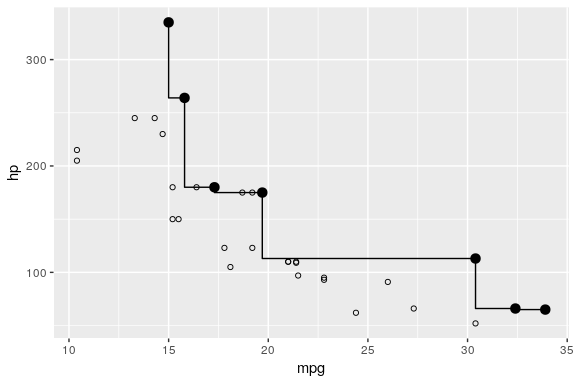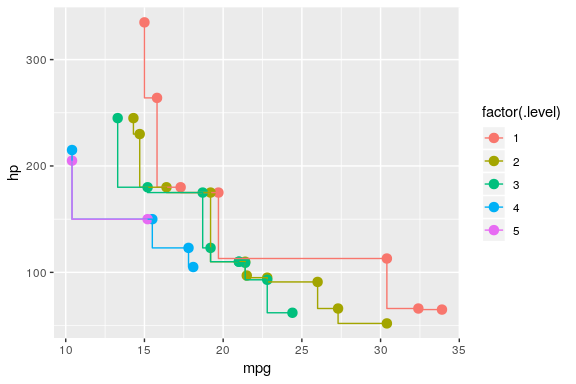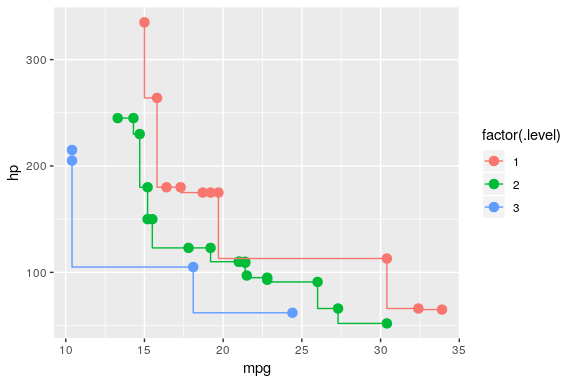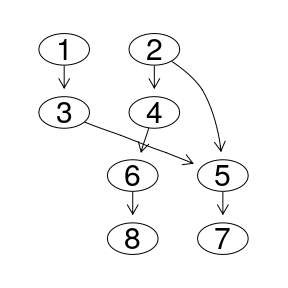# Visualization of Preferences

#### 2019-02-16

In this vignette we show methods to visualize a preference on a given data set. There are two main visualization methods:

• Skyline plots, where the stair-shaped Pareto front is highlighted. Typically this is only appropriate for 2-dimensional Skylines.

• Better-Than-Graphs, where the preference (mathematically a strict order) order on a given data set is shown as a graph. This is well suited for all kinds of preferences, but the visualized data set has to be sufficiently small.

## A first Skyline plot

For the following Skyline plots we rely on ggplot2. We get the Pareto-optimal cars with low fuel consumption and high power using the preference selection psel. We highlight them in an mpg/hp diagram and show the Pareto front line via geom_step from ggplot2.

sky <- psel(mtcars, high(mpg) * high(hp))

ggplot(mtcars, aes(x = mpg, y = hp)) + geom_point(shape = 21) +
geom_point(data = sky, size = 3) + geom_step(data = sky, direction = "vh")## Skyline level plots

Next we want to highlight all the levels (i.e., Skyline iterations to get a certain tuple) for the entire data set. To get the levels of all tuples from the data set we perform a top-k selection where k equals the number of tuples in the data set (i.e., nrow(...)):

p <- high(mpg) * high(hp)
res <- psel(mtcars, p, top = nrow(mtcars))

Now we visualize the level number using different colors and show the according Pareto front line for each level.

ggplot(res, aes(x = mpg, y = hp, color = factor(.level))) +
geom_point(size = 3) + geom_step(direction = "vh")Some segments of the Pareto front line overlap, as the Pareto order requires strict dominance in only one dimension. In the other dimensions non-strict dominance (better/equal) suffices. We replace * by the intersection operator | requiring strict dominance in both dimensions.

res <- mtcars %>% psel(high(mpg) | high(hp), top = nrow(mtcars)) %>%
arrange(mpg, -hp)

ggplot(res, aes(x = mpg, y = hp, color = factor(.level))) +
geom_point(size = 3) + geom_step(direction = "vh")In the consequence no line segments are overlapping. The number of levels reduces from 5 to 3. This visually shows the difference of Pareto-composition and the intersection preference (mathematically the product order). Note that arrange (from dplyr) is required to get the tuples with equivalent values in one of the dimensions in the correct order to avoid U-shaped lines in the stair-shaped Pareto front line.

## Better-Than-Graphs

The Better-Than-Graph (BTG) visualizes the preference order, where edges point from better tuples to worse tuples. Formally this is a Hasse diagram of the order, i.e., the transitive reduction.

Consider the following preference where we search for cars with manual transmission and many gears (in lexicographical order) and for a high mpg value (Pareto-composed), i.e.,

p <- (true(am == 1) & high(gear)) * high(mpg)

We pick the cars from the four first levels and add the row number to the data set (the row numbers are the default labels for plotting).

df <- psel(mtcars, p, top_level = 4)
df\$num <- 1:nrow(df)
knitr::kable(select(df, num, am, gear, mpg, .level))
num am gear mpg .level
Toyota Corolla 1 1 4 33.9 1
Lotus Europa 2 1 5 30.4 1
Fiat 128 3 1 4 32.4 2
Porsche 914-2 4 1 5 26.0 2
Honda Civic 5 1 4 30.4 3
Ferrari Dino 6 1 5 19.7 3
Fiat X1-9 7 1 4 27.3 4
Ford Pantera L 8 1 5 15.8 4

We use plot_btg to generate the Better-Than-Graph. This uses Rgraphviz and the dot layouter when available (the Rgraphviz package is only available on Bioconductor) and igraph otherwise. In general, the dot layouter is more appropriate for strict orders and generates better layouts. It ensures that all edges are pointing from top to bottom. Note that the layout looks not very pretty if Rgraphviz is not available.

plot_btg(df, p)The tuples having the same level are placed on the same row. Here we have 4 rows, corresponding to the 4 levels which were selected using top_level = 4. The label of each node corresponds to the row number of the data frame, i.e., the num column in this case.

## Predecessors and successors in the BTG

In addition to the visualization, rPref offers functions to explore the predecessors and successors of the Better-Than-Graph. First we have to associate the preference with the given data set and initialize the predecessor/successor functions. This initialization internally calculates all Better-Than-Relations on the data set.

assoc.df(p) <- df
init_pred_succ(p)

Now we can obtain some worse/better tuples w.r.t. the preference order. Considering again the result of plot_btg(df, p) (where the labels are the row numbers) we have a closer look at the node 5. We get all predecessors of 5 with:

all_pred(p, 5)
##  1 2 3

We see that this result coincides with the plotted graph. Edges from the nodes 1, 2 and 3 are pointing to node 5, which means that these cars are better than the car with row number 5 according to the given preference. We can get the direct predecessors of tuple 5 by using the hasse_pred function. The resulting tuples are connected to tuple 5 with exactly one edge, i.e., are the predecessors in the Hasse diagram (transitive reduction).

hasse_pred(p, 5)
##  2 3

It is also possible to call the predecessor/successor function with a set of tuples. By default, the union of the predecessors/successors is returned. For instance, to get the union of all predecessors of both nodes 5 and 6 we call all_pred with a vector c(5, 6):

all_pred(p, c(5, 6))
##  1 2 3 4

Finally the intersection of a set of predecessors is obtained using the additional parameter intersect = TRUE. As we also see in the Better-Than-Graph the only tuple in the intersection is 2:

all_pred(p, c(5, 6), intersect = TRUE)
##  2